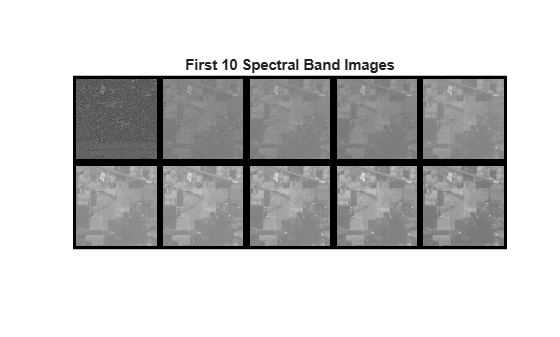# hypermnf

Maximum noise fraction transform of hyperspectral data

## Syntax

``outputDataCube = hypermnf(inputData,numComponents)``
``[outputDataCube,coeff] = hypermnf(inputData,numComponents)``
``[___] = hypermnf(inputData,numComponents,'MeanCentered',flag)``

## Description

example

````outputDataCube = hypermnf(inputData,numComponents)` computes specified number of principal component bands `numComponents` by using the maximum noise fraction (MNF) transform. To achieve spectral dimensionality reduction, the specified number of principal components must be less than the number of spectral bands in the input data cube.The components derived using MNF transform are also called non-adjusted principal components and the MNF transform arranges principal components (PC) in the decreasing order of PC image quality.```

example

````[outputDataCube,coeff] = hypermnf(inputData,numComponents)` also returns the MNF coefficients estimated across the spectral bands of the input data cube.```
````[___] = hypermnf(inputData,numComponents,'MeanCentered',flag)` computes MNF transform from mean centered spectral bands. The option for mean centering each spectral band in the input data cube is specified by `flag`. NoteThis function requires the Image Processing Toolbox™ Hyperspectral Imaging Library. You can install the Image Processing Toolbox Hyperspectral Imaging Library from Add-On Explorer. For more information about installing add-ons, see Get and Manage Add-Ons. ```

## Examples

collapse all

Read a hyperspectral data into the workspace.

`hcube = hypercube('indian_pines.dat');`

Compute 10 principal component bands of hyperspectral data and the associated transformation coefficients.

`[outputDataCube,coeff] = hypermnf(hcube,10);`

Display the first 10 spectral bands in input data cube.

```figure montage(hcube.DataCube(:,:,1:10),'BorderSize',[10 10],'Size',[2 5],'DisplayRange',[]); title('First 10 Spectral Band Images')```For the purpose of visualization, rescale the principal component values to lie in the range [0, 1]. Display all the principal component bands extracted from the data cube. The principal component bands are arranged in the order of decreasing image quality (or increasing noise level).

```figure rescalePC = rescale(outputDataCube,0,1); montage(rescalePC,'BorderSize',[10 10],'Size',[2 5]); title('Principal Component Bands of Data Cube')```## Input Arguments

collapse all

Input hyperspectral data, specified as a 3-D numeric array that represent the hyperspectral data cube of size M-by-N-by-C or `hypercube` object. If the input is a `hypercube` object, the function reads the data cube stored in the `DataCube` property of the object. The hyperspectral data cube must be real and non-sparse.

Data Types: `single` | `double` | `int8` | `int16` | `int32` | `int64` | `uint8` | `uint16` | `uint32` | `uint64`

Number of principal component bands to extract from the data cube, specified as a positive integer scalar. The value must be less than or equal to the number of spectral bands in the input data cube.

Data Types: `single` | `double` | `int8` | `int16` | `int32` | `int64` | `uint8` | `uint16` | `uint32` | `uint64`

Indicator for mean centering spectral bands, specified as one of these values:

• `true` or `1` — Mean center each spectral bands in the input data cube by subtracting the mean of spectral bands before computing the MNF transform.

• `false` or `0` — Compute principal component bands without mean centering the spectral bands in the input data cube.

Data Types: `logical`

## Output Arguments

collapse all

MNF transformed data cube, returned as a 3-D numeric array of size M-by-N-by-numComponents. The spatial dimension of the output data cube is same as that of the input data cube. The spectral dimension of the output data cube is equal to the number of principal components specified at the input.

If the input data type is double, the output data type is also double. Otherwise, the output data type is single.

Data Types: `single` | `double`

MNF coefficients, returned as a matrix of size C-by-numComponents. C is the number of spectral bands in the input data cube. Each column of `coeff` contains the coefficients for one principal component. The columns are in the order of principal component image quality.

If the input data type is double, the data type of `coeff` is also double. Otherwise, the data type is single.

Data Types: `single` | `double`

 Green, A.A., M. Berman, P. Switzer, and M.D. Craig. “A Transformation for Ordering Multispectral Data in Terms of Image Quality with Implications for Noise Removal.” IEEE Transactions on Geoscience and Remote Sensing 26, no. 1 (January 1988): 65–74. https://doi.org/10.1109/36.3001.